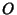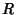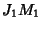## Excenter

The centerof an Excircle. There are three excenters for a given Triangle, denoted,,. The Incenterand excentersof a Triangle are an Orthocentric System.whereis the Circumcenter,are the excenters, andis the Circumradius (Johnson 1929, p. 190). Denote the Midpoints of the original Triangle,, and. Then the lines,, andintersect in a point known as the Mittenpunkt.

See also Centroid (Orthocentric System), Excenter-Excenter Circle, Excentral Triangle, Excircle, Incenter, Mittenpunkt

References

Dixon, R. Mathographics. New York: Dover, pp. 58-59, 1991.

Johnson, R. A. Modern Geometry: An Elementary Treatise on the Geometry of the Triangle and the Circle. Boston, MA: Houghton Mifflin, 1929.